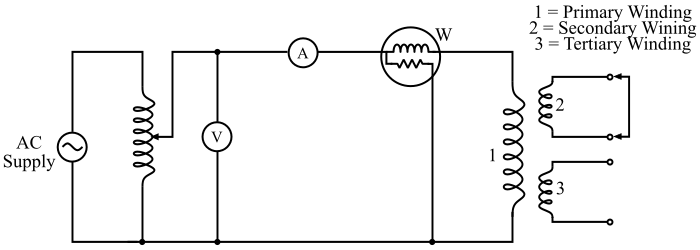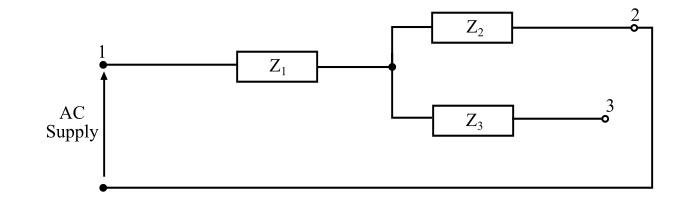# Short-Circuit Test and Open-Circuit Test on a Three-Winding Transformer

Short-circuit and open-circuit tests are to be performed on a 3-winding transformer to determine the parameters of the equivalent circuits of it.

## Short-Circuit Test

The equivalent impedances Z1, Z2 and Z3 referred to a common base can be determined by performing three short-circuit tests as follows −### Test 1

In the first test, the secondary winding is short circuited, the tertiary winding is kept open circuited and a low voltage being applied to the primary winding so that full-load current flows in the secondary winding (see the figure above). Then, the voltage, current and power input to the primary winding is to be measured.

Let V1, I1 and P1 be the voltmeter, ammeter and wattmeter readings respectively. Now, if Z12 is the short circuit impedance of primary and secondary windings with tertiary winding open. Then,

$$\mathrm{Equivalent\:impedance,\:ūĚĎć_{12} =\frac{ūĚĎČ_{1}}{ūĚźľ_{1}}}$$

$$\mathrm{Equivalent\:resistance,\:ūĚĎÖ_{12} =\frac{ūĚĎÉ_{1}}{{ūĚźľ^2}_{1}}}$$

And,

$$\mathrm{Equivalent\:leakage\:reactance,\:ūĚĎč_{12} =\sqrt{{ūĚĎć^2}_{12} − {ūĚĎÖ^2}_{12}}}$$From the equivalent circuit it can be seen that the impedance Z12 is a series combination of Z1 and Z2, i.e.,

$$\mathrm{ūĚĎć_{12} = ūĚĎÖ_{12} + ūĚĎóūĚĎč_{12} = ūĚĎć_{1} + ūĚĎć_{2} … (1)}$$

### Test 2

In the second test, the tertiary winding is short circuited and the secondary winding is kept open. A low voltage is applied to the primary winding, which circulates full-load current in the tertiary winding. If Z13 is the short-circuit impedance of primary and tertiary windings with secondary winding open, then,

$$\mathrm{ūĚĎć_{13} = ūĚĎć_{1} + ūĚĎć_{3} … (2)}$$

### Test 3

In the third test, the tertiary winding is short circuited and the primary winding is kept open. A low voltage is applied to the secondary winding so that full-load current flows in the short circuited tertiary winding. If Z23 is the short circuit impedance of secondary and tertiary windings with primary open, then,

$$\mathrm{ūĚĎć_{23} = ūĚĎć_{2} + ūĚĎć_{3} … (3)}$$

Now, from the eqns. (1), (2) and (3), the impedances Z1, Z2 and Z3 can be obtained (all should be referred to primary side) as follows −

$$\mathrm{ūĚĎć_{1} =\frac{1}{2}(ūĚĎć_{12} + ūĚĎć_{13} − ūĚĎć_{23}) … (4)}$$

$$\mathrm{ūĚĎć_{2} =\frac{1}{2}(ūĚĎć_{23} + ūĚĎć_{12} − ūĚĎć_{13}) … (5)}$$

$$\mathrm{ūĚĎć_{3} =\frac{1}{2}(ūĚĎć_{13} + ūĚĎć_{23} − ūĚĎć_{12}) … (6)}$$

Where,

$$\mathrm{ūĚĎć_{1} = ūĚĎÖ_{1} + ūĚĎóūĚĎč_{1}; ūĚĎć_{2} = ūĚĎÖ_{2} + ūĚĎóūĚĎč_{2}; ūĚĎć_{3} = ūĚĎÖ_{3} + ūĚĎóūĚĎč_{3}}$$

Note – The impedances Z12 and Z13 are referred to primary side since the instruments are connected in the primary side. The impedance Z23 is referred to secondary side. Hence, it has to be referred to the primary side and only then eqns. (4), (5) & (6) can be used for calculating Z1, Z2 and Z3.

## Open-Circuit Test

The open circuit test on a three winding transformer is performed to determine the core loss, magnetising impedance and turn ratios. In the open circuit test, the primary winding is energized with both secondary and tertiary windings on open circuit. Then, we get,

$$\mathrm{Voltage\:ratio\:between\:primary\:and \:secondary ,\:ūĚźĺ_{12} =\frac{ūĚĎČ_{1}}{ūĚĎČ_{2}}}$$

$$\mathrm{Voltage\:ratio\:between\:primary\:and \:tertiary ,\:ūĚźĺ_{13} =\frac{ūĚĎČ_{1}}{ūĚĎČ_{3}}}$$

$$\mathrm{Voltage\:ratio\:between\:secondary \:and\:tertiary ,\:ūĚźĺ_{23} =\frac{ūĚĎČ_{2}}{ūĚĎČ_{3}}=\frac{ūĚĎČ_{2}/{ūĚĎČ_{1}}}{ūĚĎČ_{3}/{ūĚĎČ_{1}}}=\frac{ūĚźĺ_{13}}{ūĚźĺ_{12}}}$$